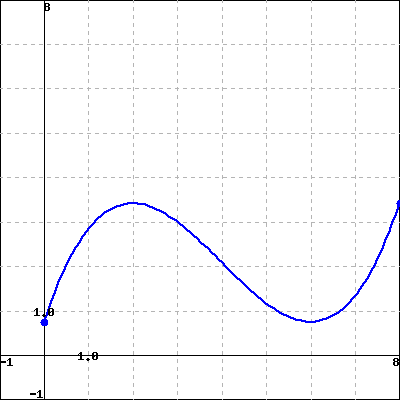A particle is moving along a horizontal straight line. The graph of the position function (the distance to the right of a fixed point as a function of time) is shown below. Answer the following questions only on the interval $(0,8)$.1. When is the particle moving toward the right?

2. When is the particle moving toward the left?